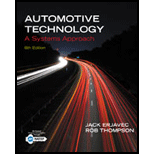Chapter 3, Problem 3RQAutomotive Technology: A Systems A...

6th Edition
Jack Erjavec + 1 other
ISBN: 9781133612315

Solutions

Chapter
SectionAutomotive Technology: A Systems A...

6th Edition
Jack Erjavec + 1 other
ISBN: 9781133612315
Textbook Problem

Explain Newton’s second law of motion and give an example of how this law is used in automotive theory.

To determine

Newton's second law of motion with an example in terms of automotive theory.

Explanation

Given Information:

It can be expressed mathematically.

F = ma

Acceleration is produced when an unbalanced force acts on an object.

The second law of motion can be expressed another way that the rate of change of momentum is known as force.

Assume that a car is stationary position and have a mass, now a ...

Still sussing out bartleby?

Check out a sample textbook solution.

See a sample solution

The Solution to Your Study Problems

Bartleby provides explanations to thousands of textbook problems written by our experts, many with advanced degrees!

Get Started

What is a buffer overflow, and how is it used against a Web server?

Principles of Information Security (MindTap Course List)

Convert P = 5.00 atm into Pa, bar, and psia.

Fundamentals of Chemical Engineering Thermodynamics (MindTap Course List)

What strategies e Wal-Mart and Loweâ€™s using to gain more online customers?

Systems Analysis and Design (Shelly Cashman Series) (MindTap Course List)